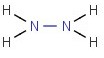# Problem: What are the molecular and empirical formulas for each of the following compounds?Write the empirical formula for the following compound.

###### FREE Expert Solution

Count the number of each of the elements to get the molecular and empirical formula.

79% (162 ratings)###### Problem Details

What are the molecular and empirical formulas for each of the following compounds?

Write the empirical formula for the following compound.Frequently Asked Questions

What scientific concept do you need to know in order to solve this problem?

Our tutors have indicated that to solve this problem you will need to apply the Empirical Formula concept. You can view video lessons to learn Empirical Formula. Or if you need more Empirical Formula practice, you can also practice Empirical Formula practice problems.